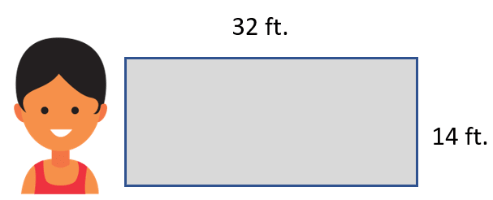## Area of Rectangles In Word Problems

#### Grade 3 Measurement - 3.MD.8

This is one of those topics you are going to wish you remembered when you get a little older. While these may seem like your typical everyday word problems, they are very relevant to all different forms of modern construction. The goal of this section is to help you to learn how to identify the object of interest and the dimensions of its structure. Students learn how to tackle a word problem that involves finding the area of a rectangle with this worksheet series.

### Printable Worksheets And Lessons#### Homework Sheets

If you do any outdoor work, you will run into this skill often.

• Homework 1- JoAnne plants a 3-foot row of flowers that takes up 10 feet. If she plants 5 rows right next to each other, what will be the perimeter of the garden?
• Homework 2- Rectangle A and B have equal areas.
• Homework 3- Find the area and perimeter of each rectangle.

#### Practice Worksheets

Figure out Joe's landscaping charges and some missing sides of rectangles.

• Practice 1- Joe's landscaping service charges customers by the square footage. Each year, customers pay \$1 per square foot of yard that Joe maintains. Calculate how much each customer owes Joe below.
• Practice 2- For 7 and 8 both rectangles have the same area. Find the value of r.

#### Math Skill Quizzes

The word problems really do trip up a lot of different people.

• Quiz 1- Jason makes book cases. The shelves of the latest book case he created holds books that are a maximum of 18 inches high. The book case has 5 shelves. Jason places a box on top of the book case. How high up is that box?
• Quiz 2- Find the missing side (x) of the rectangles.

### Example Problem

Ziggy Snow Removal Service charges \$0.50 per square foot to shovel driveways after large snow falls. Mrs. Nevens would like to have her driveway shoved by Ziggy. Based on the diagram of her driveway below, how much will it cost to have Ziggy’s service shovel it for her.#### Solution to Problem

The driveway is in the shape of a rectangle. To solve this problem, we will need to determine the area of driveway and then multiple that by Ziggy’s price per square foot.

Step 1) Determine Area: A = L x W (According to the diagram the Length = 32 ft. and Width = 14 ft.)

A = 32 ft x 14 ft = 448 ft2

Step 2) Determine Price

The total cost will be the area (square footage) multiplied by the cost per square foot. Looking back at our solution, in step 1, the square footage is 448 ft2. Looking back at the original problem the cost is \$3 per square foot. So the total cost will be = 448 x \$0.50 = \$224.

### When Is It Important to Know How Calculate the Area of a Rectangle?

A rectangle is a four-sided figure with parallel equal sides. If you look around, you’ll see so many objects in the shape of a rectangle, the most basic being a table, a carpet, and a box which are everyday objects typically seen in offices and at homes. In fact, most items constructed by people take on this shape.

In geometry, an important calculation that you can do is find the area of the rectangle. But why is it important? What is the shape of the walls you are in? What is the shape of your computer screen? Are these things in a rectangle shape? Do you see any paint or carpet around you? If you do, then you need to know that the area of the rectangle was used for figuring out how much paint would be required for a particular area on the floor.

You must know how to calculate the area of a rectangle, and the best part is, it is very simple — just multiple lengths of the rectangle with the width.

Area = Length x width - Rectangles are important in all walks of life, and therefore, you need to be able to calculate the area of the rectangle.